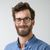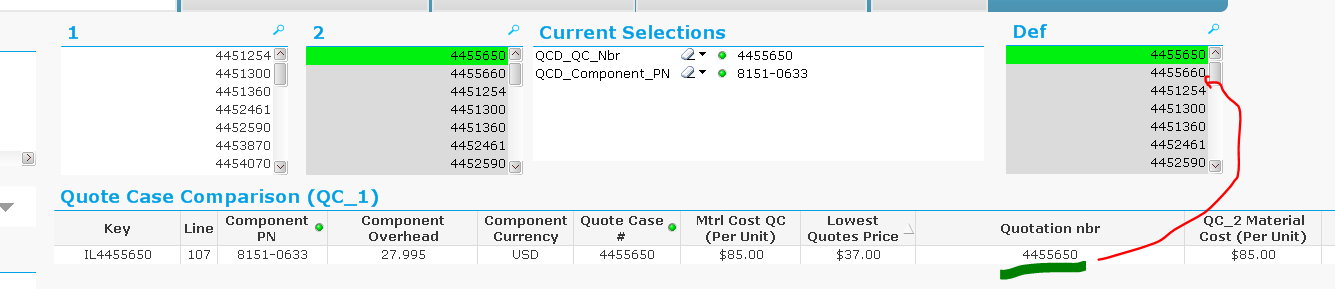# QlikView App Dev

Discussion Board for collaboration related to QlikView App Development.

Announcements
Our next Qlik Insider session will cover new key capabilities. Join us August 11th REGISTER TODAY
cancel
Showing results for
Did you mean:Contributor II

## Straight Table - how to get the cheapest vendor reference

Hello,

i've got this kind of data set:

 QCD_Material_Cost (Per Unit) QCD_QC_Nbr QCD_Component_PN Lowest Quotes Price Quotation nbr 52 4452590 52 4452590 0100-2510 52 4452590 97 4459110 0100-2510 52 256 4458800 0100-2510 52 366 4456651 0100-2510 52 386 4458790 4985-4444 52 4458790 561 4453870 4985-4444 52

i've got the lowest price and the quote number with the following expressions:

Lowest Quotes Price: min(TOTAL <QCD_Component_PN> [QCD_Material_Cost (Per Unit)])

Quotation nbr: concat( aggr(if(min([QCD_Material_Cost (Per Unit)])=min(total <QCD_Component_PN> [QCD_Material_Cost (Per Unit)]),QCD_QC_Nbr),QCD_QC_Nbr,QCD_Component_PN),', ')

i woudl like that the  Quotation nbr of the lowest price will be listed for the other lines too.

so i will get this table

 CD_Material_Cost (Per Unit) QCD_QC_Nbr QCD_Component_PN Lowest Quotes Price Quotation nbr 52 4452590 52 4452590 0100-2510 52 4452590 97 4459110 0100-2510 52 4452590 256 4458800 0100-2510 52 4452590 366 4456651 0100-2510 52 4452590 386 4458790 4985-4444 52 4458790 561 4453870 4985-4444 52 4458790

Thanks, Barak

17 RepliesContributor II
Author

concat(total <QCD_Component_PN> aggr(if(min([QCD_Material_Cost (Per Unit)])=min(total <QCD_Component_PN> [QCD_Material_Cost (Per Unit)]),QCD_QC_Nbr),QCD_QC_Nbr,QCD_Component_PN),', ')

add the total qualifier on the concatContributor III

concat(total <QCD_Component_PN> aggr(if(min([QCD_Material_Cost (Per Unit)])=min(total <QCD_Component_PN> [QCD_Material_Cost (Per Unit)]),QCD_QC_Nbr),QCD_QC_Nbr,QCD_Component_PN),', ')Champion II

Now I see what you need, just use the below

=FirstSortedValue(TOTAL <QCD_Component_PN> QCD_QC_Nbr,QCD_Material_Cost)Creator III

Punit's answer would be much simpler and straight forward to use instead of concate(...) oneContributor II
Author

Hello Purjari, in case 2 vendors has minmum price it will return only 1 of them

thanks, BarakChampion II

You can tweak the expression like below

= FirstSortedValue(TOTAL<QCD_Component_PN> AGGR(concat(DISTINCT QCD_QC_Nbr,','),QCD_Material_Cost) , aggr(QCD_Material_Cost,QCD_Material_Cost))Contributor II
Author

Hello Pujari,

your expression responds to user selections, can you please advise how to implement for global implemanation ?

the number on the second table should be 44555660

Thanks!

= FirstSortedValue(total <QCD_Component_PN>AGGR(concat( QCD_QC_Nbr,','),[QCD_Material_Cost (Per Unit)]) , aggr([QCD_Material_Cost (Per Unit)],[QCD_Material_Cost (Per Unit)]))Contributor II
Author

Hello Amir,

your exspression has the same issue, its sensetive to user selections and does not provides with global answer.

(see my respond to Punit)

ThanksChampion II

Use below

= FirstSortedValue({1}TOTAL<QCD_Component_PN> AGGR(concat({1}DISTINCT QCD_QC_Nbr,','),QCD_Material_Cost) , aggr(QCD_Material_Cost,QCD_Material_Cost))Community Browser